# University of Wisconsin Green Bay

An archer shoots an arrow at a 22o angle to the horizontal. At the top of its motion, the arrow just clears a 36 m high oak tree. How fast was the arrow going when it left the bow? (Assume it was shot from a height of 1.0 m above the ground.) How far away is the archer from the tree?

• In this problem, you are asked to describe the motion (how fast does it fly, how far does it go) of an arrow. Whenever you are asked to describe the motion of an object without worrying about the cause of that motion, you have a kinematics problem.

•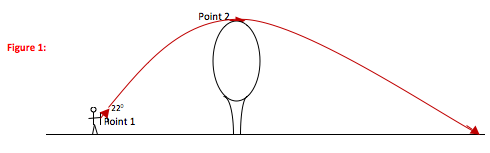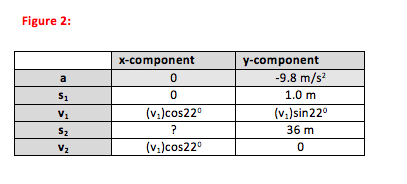•There are three key kinematic equations. If you carefully select the equation which most directly describes the situation in your problem, you will not only solve the problem in fewer steps but also understand it better. The three equations, written for motion in the y-direction, are:

1. y = y0 + v0yΔt + ½ ay(Δt)2 (relates position and time)

2. vy = v0y + ayΔt (relates velocity and time)

3. vy2 = v0y2 + 2ay(Δy) (relates velocity and position)

a) In this problem, you are asked for the initial velocity of the arrow. Initial velocity shows up in all three equations, and so it will not help you select the most useful equation to use. However, you know you want to find the initial velocity that results in the arrow reaching the top of its motion (y-velocity = 0) when it is at a height (y-position) of 36 m. So you will use equation 3 in the y-direction.

b)You are also asked how far the tree is from the archer (x-position). The x-position that you want is the one that corresponds to a y-velocity of zero. Remember, you cannot mix x- and y-components in a single equation.

Whenever you are asked about the x-direction and told about the y-direction, or vice versa, you need to use time to go between the two.

The amount of time that the arrow spends going from the bow to its maximum height (vy = 0) is the same as the amount of time it takes to go from the bow to the tree. So you can use what you know about the y-velocity of the ball to find the time it spends in the air (equation 2 in the y-direction) and then use that time to find its x-position (equation 1 in the x-direction.)

• Step 1: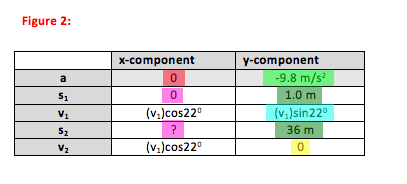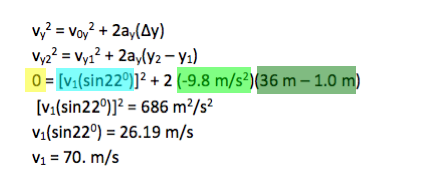You have answered the first question: the arrow leaves the bow at a speed of 70. m/s. Scroll down to solve for the distance between the archer and the tree.

--------------------------------------------------------------------------------------------

Step 2: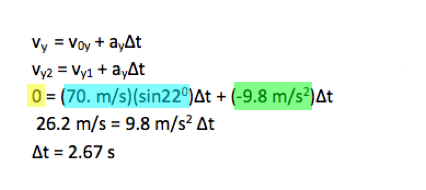Remember that Δt refers to the time between the two points put into the equation. So it takes the arrow 2.7 s to go from the bow to the tree. Now that you know the time it took to cover this distance, scroll down to calculate the distance.

--------------------------------------------------------------------------------------------

Step 3: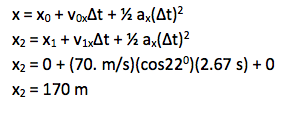The problem asked you to find the horizontal (x) distance between the archer (Point 1) and the tree (Point 2.) No further mathematical solution is necessary.

•In this problem, you are asked to find how fast an arrow needs to travel in order to reach a maximum (y-velocity = 0) height of 36 m. Therefore, regardless of the unknown quantity, the most direct relationship for the problem is the position-velocity equation in the y-direction.

Next, you are asked how far the arrow travels during that time. Displacement, velocity and acceleration are vector quantities, and so you cannot mix x- and y- components in the same equation. Therefore, you cannot use the y-velocity condition (vy2 = 0) to solve for the x-position question (how far away is the tree) in a single step.

You can always relate x- and y-motion through time—the arrow spends as long going up as it spends going from the archer to tree. So we first solved for time required to go up to a maximum point (y-velocity) and then used that time to determine how far the ball traveled (x-position.)